﻿ 抽水蓄能电站上下库渗流场数值分析 Numerical Analysis of Seepage Field in the Upper and Lower Reservoirs of Pumped Storage Power Station

Journal of Water Resources Research
Vol.06 No.06(2017), Article ID:22248,7 pages
10.12677/JWRR.2017.66074

Numerical Analysis of Seepage Field in the Upper and Lower Reservoirs of Pumped Storage Power Station

Jiahuan He, Yue Zhang

State Key Laboratory Base of Eco-Hydraulic Engineering in Arid Area, Xi’an University of Technology, Xi’an Shaanxi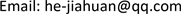Received: Sep. 6th, 2017; accepted: Sep. 22nd, 2017; published: Sep. 30th, 2017ABSTRACT

The theoretical basis of numerical analysis of seepage field is reviewed. Based on the geological and hydrogeological conditions of upper and lower reservoirs of a pumped storage power station, the three-dimensional numerical simulation model of dam was established by using ADINA finite element software. The distributions of seepage and seepage field of upper and lower reservoirs under various operating conditions were calculated and analyzed. Under the condition of changing the water level of the upper reservoir, the variation law of the seepage parameters in the reservoir water level is analyzed, and the change of the free surface of the dam is calculated. The water level decreases, such as the head line, the infiltration line and seepage flow. It is concluded that the seepage flow is decreasing but the infiltration line remains essentially unchanged during the descent of the water level.

Keywords:Pumped Storage Power Station, Seepage Field, Upper and Lower Reservoir, ADINA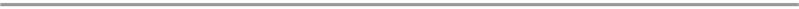Copyright © 2017 by authors and Hans Publishers Inc.1. 引言

2. 渗流的基本方程

$\frac{\partial }{\partial x}\left({k}_{x}\frac{\partial H}{\partial x}\right)+\frac{\partial }{\partial y}\left({k}_{y}\frac{\partial H}{\partial y}\right)+\frac{\partial }{\partial z}\left({k}_{z}\frac{\partial H}{\partial z}\right)+\omega =0$ (1)

${H\left(x,y,z\right)|}_{S1}=\varphi \left(x,y,z\right)\text{\hspace{0.17em}}\text{\hspace{0.17em}}\text{\hspace{0.17em}}\left(x,y,z\right)\in S1$ (2)

${k\frac{\partial H}{\partial n}|}_{S2}=q\left(x,y,z\right)\text{\hspace{0.17em}}\text{\hspace{0.17em}}\text{\hspace{0.17em}}\left(x,y,z\right)\in S2$ (3)

$\left\{\begin{array}{l}\frac{\partial H}{\partial n}=0\\ {H\left(x,y,z\right)|}_{S3}=Z\left(x,y\right)\text{\hspace{0.17em}}\text{\hspace{0.17em}}\text{\hspace{0.17em}}\left(x,y,z\right)\in S3\end{array}$ (4)

$\left\{\begin{array}{l}\frac{\partial H}{\partial n}<0\\ {H\left(x,y,z\right)|}_{S4}=Z\left(x,y\right)\text{\hspace{0.17em}}\text{\hspace{0.17em}}\text{\hspace{0.17em}}\left(x,y,z\right)\in S4\end{array}$ (5)

3. 工程概况

4. 算例分析

4.1. 计算模型与材料参数

(1) 左侧、右侧、上游及下游边界：根据长期观测资料，反演算计算天然渗流场，边界上水位选为天然地下水位。

(2) 模型的底部，上水库模型底面高程为250 m，下水库模型底面高程为150 m，即至坝基开挖面下约为三倍坝高，上水库顶部地表最高高程为762.76 m，下水库顶部地表最高高程为369.04 m，都位于较深的基岩内，因此，该边界面上取为隔水边界。

(3) 上水库库内：取为自由逸出边界；下水库库内：施工期取为自由逸出边界。

(4) 对于水库其他主要建筑物，比如压力管道、尾水隧洞、排水廊道及排水孔、电站厂房等在模型建立中概化处理。

(5) 坝体以及坝基各个材料渗透系数如表2所示，将表中的材料各个参数添加到渗流模型中进行渗流场计算。所得到的上水库和下水库模型分别如图1所示。Table 1. Main engineering characteristics of a pumped storage power stationTable 2. Permeability coefficient of each partition material

4.2. 正常蓄水位下的渗流场分析

4.3. 库水位下降的渗流场分析

5. 结语

(1) 通过结合某抽水蓄能电站上下库枢纽区工程资料设定坝体各个材料的渗透系数均在实际工程范围内，具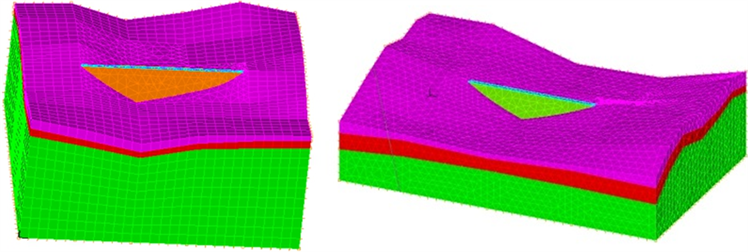Figure 1. Upper and lower reservoir models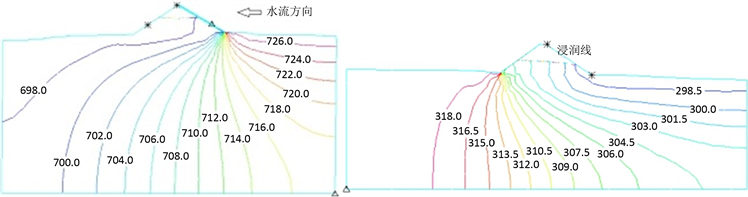Figure 2. Contour map of the total head of the largest section of the upper and lower reservoir dam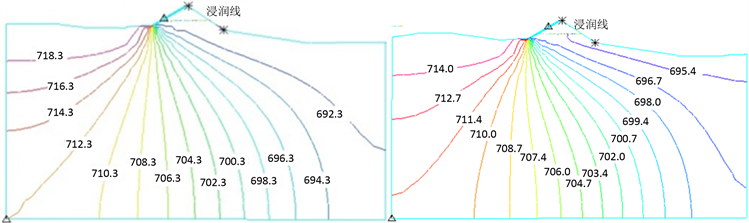(a) (b)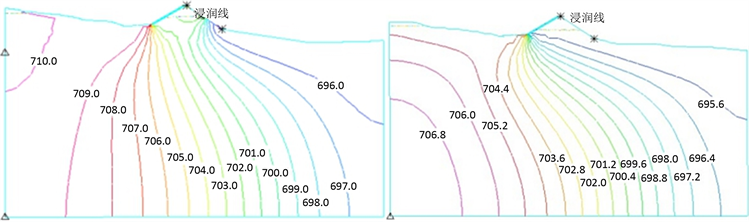(c) (d)

Figure 3. Total head contour map of the largest section when upper reservoir water level drops: (a) Water level drop 5 m total head contour map; (b) Water level drop 10 m total head contour map; (c) Water level drop 15 m total head contour map; (d) Water level drop 20 m total head contour map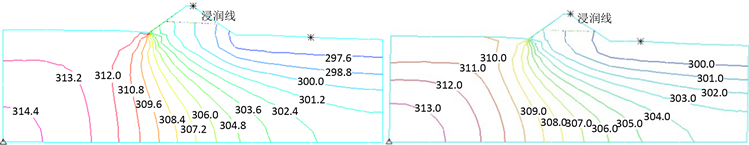(a) (b)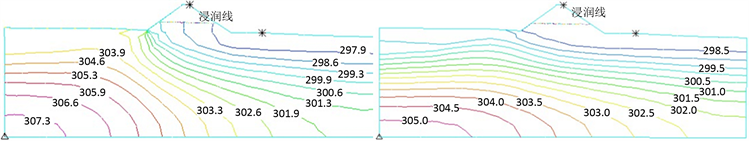(c) (d)

Figure 4. Total head contour map of the largest section when down reservoir water level drops: (a) Water level drop 5 m total head contour map; (b) Water level drop 10 m total head contour; (c) Water level drop 15 m total head contour map; (d) Water level drop 20 m total head contour map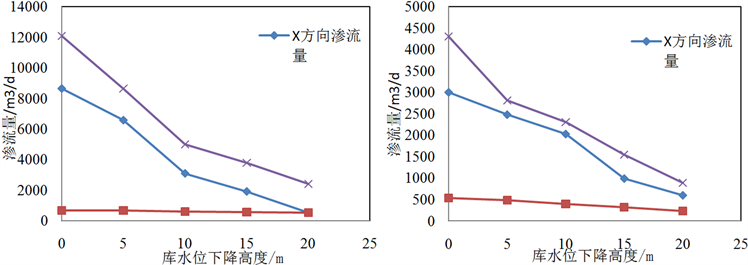Figure 5. Upper and lower reservoir dam water level drop seepage flow

(2) 在上水库和下水库水位不断下降的情况下，库水位的变化基本不会引起坝体内部浸润线的变化，但是渗流量会随之减小，基本呈现线性关系。

Numerical Analysis of Seepage Field in the Upper and Lower Reservoirs of Pumped Storage Power Station[J]. 水资源研究, 2017, 06(06): 635-641. http://dx.doi.org/10.12677/JWRR.2017.66074

1. 1. 冯硕谋. 我国最大的抽水蓄能电站在建设中——广州抽水蓄能电站简介[J]. 水电站设计, 1990(4): 91-92. FENG Shuomou. A brief introduction of the Guangzhou pumped storage power station in the construction of the largest pumped storage power station in China. Hydropower Station Design, 1990(4): 91-92. (in Chinese)

2. 2. 张娜, 董化宏, 何学铭. 我国抽水蓄能电站建设情况[J]. 中国三峡科技版, 2010(11): 12-15. ZHANG Na, DONG Huahong and HE Xuemin. Construction situation of pumped storage power stations in China. China Three Gorges Science and Technology Edition, 2010(11): 12-15. (in Chinese)

3. 3. 任涵璐, 刘斯宏, 姜忠见, 等. 洪屏抽水蓄能电站上水库库盆三维复杂渗流场分析[J]. 水力发电, 2012, 38(12): 19-21. REN Hanlu, LIU Sihong, JIANG Zhongjian, et al. 3-D Seepage field analysis for upper reservoir basin of Hongping pumped-storage station. Water Power, 2012, 38(12): 19-21. (in Chinese)

4. 4. 吴海亮, 牛贺道, 张有山. 回龙抽水蓄能电站上库渗流模拟计算与防渗新工艺[J]. 水利水电科技进展, 2006(1): 54-56+69. WU Hailiang, NIU Daohe, ZHANG Youshan. Simulation calculation and new technique for upper layer of Huilong pumped storage power station. Advances in Science and Technology of Water Resources, 2006(1): 54-56+69. (in Chinese)

5. 5. 李平, 卢文喜, 李俊, 杨威. 蒲石河抽水蓄能电站上库三维渗流数值模拟[J]. 水文地质工程地质, 2008, 35(2): 95-98. LI Ping, LU Wenxi, LI Jun, YANG Wei. Numerical simulation of three-dimensional seepage flow in upper reservoir of Pu-shihe pumped storage power station. Hydrogeology & Engineering Geology, 2008, 35(2): 95-98. (in Chinese)

6. 6. 孙立昌. 仙游抽水蓄能电站上下库大坝蓄水期运行状况分析[J]. 中国水能及电气化, 2015(2): 36-39. SUN Lichang. Analysis on operation condition of reservoir dam of Xianyou pumped storage power station. China Water Power & Electrification, 2015(2): 36-39. (in Chinese)

7. 7. 刘昌军, 王小卫, 徐甲存, 等. 文登抽水蓄能电站地下洞室群复杂渗流场的数值模拟分析[J]. 长江科学院院报, 2013, 30(4): 73-78. LIU Changjun, WANG Xiaowei, XU Jiacun, et al. Numerical simulation analysis of complex seepage field in underground chamber group of Wendeng pumped storage power station. Journal of Yangtze River Scientific Research Institute, 2013, 30(4): 73-78. (in Chinese)